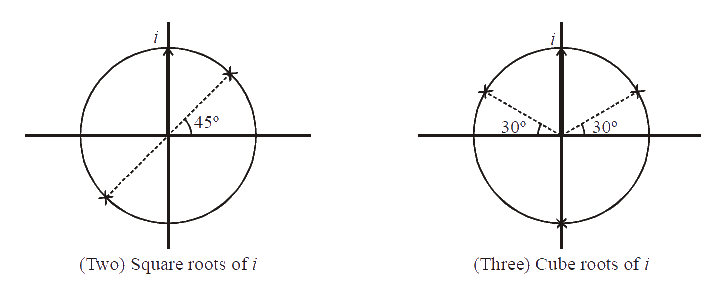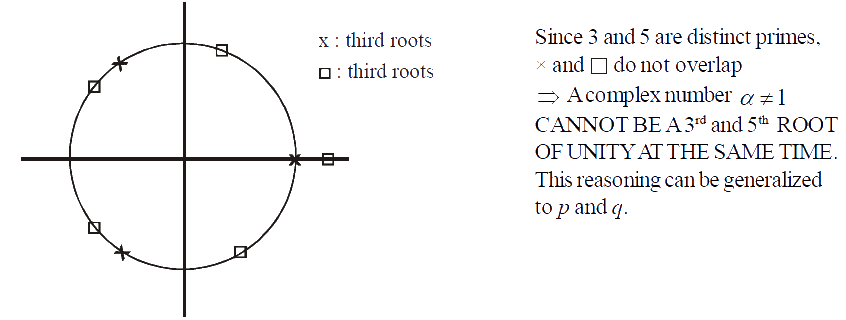# Examples On Roots Of Complex Numbers

Go back to  'Complex Numbers'

Example - 26

Find the square roots and the cube roots of i.

Solution:    First write i in its Euler’s form.

\begin{align}&i = {e^{\frac{{i\pi }}{2}}}\end{align}

Square roots:

\begin{align}&{z^2} = {e^{i\pi /2}} = {e^{i\left( {2p\pi + \frac{\pi }{2}} \right)}}\\ &\;\;\;\;\;\Rightarrow \,\,\,z = {e^{i\left( {p\pi + \frac{\pi }{4}} \right)}}\end{align}

The two roots are given by two consecutive values of p, say p = 0, 1

\begin{align}&\Rightarrow \,\,\,{z_1} = {e^{i\,\,\pi /4}},\,\,{z_2} = {e^{i\,\,5\pi /4}}\end{align}

Cube roots:

\begin{align}&\;\;\;\;\;\;{z^3} = {e^{i\left( {2p\pi + \frac{\pi }{2}} \right)}}\\ &\Rightarrow \,\,\,z = {e^{i\left( {\frac{{2p\pi }}{3} + \frac{\pi }{6}} \right)}} \end{align}

There are three roots; take \begin{align}&\Rightarrow \,\,\,{z_1} = {e^{i\,\pi /6}},\,\,{z_2} = {e^{i5\,\pi /6}},\,\,{z_3} = {e^{i3\,\pi /2}}\end{align}

Let us plot the square roots and cube roots of i on the plane:Example - 27

If $$1,\,\,{\alpha _1},\,\,{\alpha _2}...,\,\,{\alpha _{n - 1}}$$ are the $${n^{th}}$$ roots of unity, find the value of $$(1 + {\alpha _1})(1 + {\alpha _2})...(1 + {\alpha _{n - 1}})$$ .

Solution:    Since $${x^n} - 1 = 0$$ has n roots (that have been specified in the question), we can write         (by factor theorem):

\begin{align}&\qquad\;\;{x^n} - 1 = (x - 1)(x - {\alpha _1})...(x - {\alpha _{n - 1}})\\&\Rightarrow \,\,\,\frac{{{x^n} - 1}}{{x - 1}} = (x - {\alpha _1})(x - {\alpha _2})...(x - {\alpha _{n - 1}})\;\;\;\;\;\;\;....(1)\end{align}

The form of the expression whose value we need to obtain hints that we should substitute $$x = - 1$$ in (1):

\begin{align}&\frac{{{{( - 1)}^n} - 1}}{{( - 1) - 1}} = ( - 1 - {\alpha _1})( - 1 - {\alpha _2})...( - 1 - {\alpha _{n - 1}})\\\,\,\,\,\,\,\,\,\,\,\,\,\,\,\,\,\,\,\, &\qquad\quad\quad\;\;= {( - 1)^{n - 1}}(1 + {\alpha _1})(1 + {\alpha _2})...(1 + {\alpha _{n - 1}})\end{align}

If n is even the left hand side is 0 so that the value of the expression becomes 0. If n is odd, the expression becomes

\begin{align}&\,\,\,\,\,\,\,\,\,\,\,\,\,\,\,\,\,\,\,\,\,\,\,\,\frac{{ - 2}}{{ - 2}} = {( - 1)^{n - 1}}(1 + {\alpha _1})(1 + {\alpha _2})...(1 + {\alpha _{n - 1}})\\ &\;\;\;\Rightarrow \,\,\,(1 + {\alpha _1})(1 + {\alpha _2})...(1 + {\alpha _{n - 1}}) = 1\end{align}

Thus, the expression takes the value 0 or 1 depending on whether n is even or odd respectively.

Example - 28

Let $$\omega ,\,\,{\omega ^{\,2}}$$ be the complex cube roots of unity. Let $${z_{1,}}\,\,{z_2},\,\,{z_3}$$ be complex numbers such that

$\begin{array}{l}\,\,\,\,\,\,\,\,\,{z_1} + {z_2} + {z_3} = A\\{z_1} + \omega {z_2} + {\omega ^2}{z_3} = B\\{z_1} + {\omega ^2}{z_2} + \omega {z_3} = C\end{array}$

Evaluate $${z_1},\,\,{z_2}{\rm{ and }}{z_3}$$ in terms of A, B, and C.

Solution:    We know that $$1 + \omega + {\omega ^2} = 0$$

We have to use this to somehow express each of $${z_1},\,\,{z_2}{\rm{ and }}{z_3}$$ independently in terms of A, B and C. Label the three equations as (I), (II) and (III).  (I) + (II) + (III) gives

\begin{align}& \,\,\,\,\,\,\,\,\,\,\,\,3{z_1} = A + B + C\\ &\;\;\Rightarrow \,\,\,{z_1} = \frac{{A + B + C}}{3} \end{align}

$$\left( I \right){\rm{ }} + w{^2}{\rm{ }}\left( {II} \right){\rm{ }} + w\left( {III} \right){\rm{ }}gives$$

\begin{align}&\;\;\,\,\,\,\,\,3{z_2} = A + {\omega ^2}B + \omega C\\ &\Rightarrow \,\,\,{z_2} = \frac{{A + B{\omega ^2} + C\omega }}{3}\end{align}

Finally, $$\left( I \right){\rm{ }} + w{^2}{\rm{ }}\left( {II} \right){\rm{ }} + w\left( {III} \right){\rm{ }}gives$$

\begin{align}&\;\,\,\,\,\,\,3{z_3} = A + B\omega + C{\omega ^2}\\ &\Rightarrow \,\,\,{z_3} = \frac{{A + B\omega + C{\omega ^2}}}{3}\end{align}

These were the values we were looking for.

Example - 29

Let a complex number $$\alpha ,\,\,\alpha \ne 1$$ be a root of $${z^{p + q}} - {z^p} - {z^q} + 1 = 0,$$ where p and q are distinct primes. Show that either $$1 + \alpha + {\alpha ^2} + ... + {\alpha ^{p - 1}} = 0$$ or $$1 + \alpha + {\alpha ^2} + ... + {\alpha ^{q - 1}} = 0$$ , but not both together

Solution:    The given equation can be written as

$({z^p} - 1)({z^q} - 1) = 0$

Therefore, $$\alpha$$ is a root of either $${z^p} - 1 = 0\,\,{\rm{or }}{z^q} - 1 = 0$$ . In other words, $$\alpha$$ is either a $${p^{th}}$$ or a $${q^{th}}$$ root of unity.

Now,

$${z^p} - 1 = (z - 1)({z^{p - 1}} + {z^{p - 2}} + .... + z + 1)$$

Substituting $$z = \alpha$$ gives

$0 = (\alpha - 1)({\alpha ^{p - 1}} + {\alpha ^{p - 2}} + ..... + \alpha + 1)$

Since $$\alpha \ne 1,$$ we get

$1 + \alpha + {\alpha ^2} + .... + {\alpha ^{p - 1}} = 0\,\,\,\,\,\,\,\,\,\,\,\,\,\,\,\,\,\,\,\,\,\,\,\,\,\,\,\,\,\,\,...\left( 1 \right)$

Similarly, if   $${z^q} - 1 = 0,$$ we get

$1 + \alpha + {\alpha ^2} + ..... + {\alpha ^{q - 1}} = 0\,\,\, \ldots (2)$

We now need to show that (1) and (2) cannot hold simultaneously. In other words, $$\alpha$$ cannot be a $$p{^{th}}{\rm{ }}\;and\;{\rm{ }}a\;{q^{th}}$$  root of unity at the same time (given the condition that p and q are distinct primes). This is easy to prove:

If $$\alpha$$ is a p th  root of unity,

$\alpha = {e^{^{i\frac{{2m\pi }}{p}}\,\,\,\,\,\,}}\,\,\,\,\,\,\,\,\,\,\,\,\,\,\, \ldots (3)$

If $$\alpha$$ is a q th  root of unity,

$\alpha = {e^{i\frac{{2n\pi }}{q}}}\,\,\,\,\,\,\,\,\,\,\,\,\,\,\,\,\,\,\,\,\,...\left( 4 \right)$

Observe the right hand sides of (3) and (4) carefully. The terms in the exponents $$\frac{m}{p}$$ , (where m < p) and $$\frac{n}{q}$$ (where n < q) can never be equal. Why? Lets assume they are equal

\begin{align}&\,\,\,\,\,\,\,\,\,\frac{m}{p} = \frac{n}{q}\\ &\Rightarrow pn = mq\end{align}

Since p is a prime, different from q, it cannot have m and q and factors. Since n < q, n cannot have q as a factor. Thus pn cannot have m or q as factors which is a contradiction.

$$\Rightarrow$$  (3) and (4) cannot be equal

$$\Rightarrow$$  (1) and (2) cannot be simultaneously satisfied.

Here’s an example, for p = 3 and q = 5:

p=3,q=5Example - 30

Find all the roots of the equation $${z^{12}} - 56{z^6} - 512 = 0$$ whose imaginary parts are non-negative

Solution:    We let $${z^6} = x$$ so that the given equation reduces to a quadratic.

$\begin{array}{l}\,\,\,\,\,\,\,\,\,{x^2} - 56x - 512 = 0\\\,\,\,\,\,\,\,\,\,(x - 64)(x + 8) = 0\\ \Rightarrow x = 64, - 8\\ \Rightarrow {z^6} = 64, - 8\end{array}$

(a) $$\fbox{\({z^6}$$ = 64}\)

\begin{align}&{z^6} = 64 = {2^6}{e^{i(2p\pi + 0)}}\\ &\quad\;\Rightarrow z = 2{e^{i\left( {\frac{{2p\pi + 0}}{6}} \right)}}\end{align}

Verify that for p = 0, 1, 2, 3, we get non-negative imaginary parts for z:

$z = 2,\,\,\,\,2{e^{i\pi {\rm{/3}}}},\,\,\,\,2{e^{i2\pi {\rm{/3}}}},\,\,\,\,\,2{e^{i\pi }}$

(b) $$\fbox{\({z^6}$$ =  - 8}\)

$\begin{array}{l}\,\,\,\,\,\,{z^6} = - 8 = {2^3}{e^{i\pi }} = {2^3}{e^{i(2p\pi + \pi )}}\\ \Rightarrow z = {2^{1{\rm{/2}}}}{e^{i\left( {\frac{{2p\pi + \pi }}{6}} \right)}}\end{array}$

Verify that for p = 0, 1, 2, we get non-negative imaginary parts for z:

\begin{align}&z = \sqrt 2 {e^{i\pi {\rm{/6}}}},\sqrt 2 {e^{i\pi {\rm{/2}}}},\sqrt 2 {e^{i5\pi {\rm{/6}}}}\end{align}

Thus we get seven values of z that satisfy the given condition.

## TRY YOURSELF - VIII

Q. 1      By considering the eleventh roots of unity, find the value of

$S = \sum\limits_{k = 1}^{10} {} \left( {\sin \left( {\frac{{2\pi k}}{{11}}} \right) - i\cos \left( {\frac{{2\pi k}}{{11}}} \right)} \right)$

Q. 2      Find the value of $$(\alpha - 1)(\alpha - {\alpha ^2})(\alpha - {\alpha ^3})...(\alpha - {\alpha ^{n - 1}})$$ where $$\alpha = {e^{i2\pi /n}}$$ .

Q. 3      If ABC is an equilateral triangle having vertices $${z_1}{\rm{ }},{z_2}{\rm{ }}\;and\;{z_3}{\rm{ }}$$  (in the anti-clockwise direction), find the value of $${z_1} + \omega {z_2} + {\omega ^2}{z_3}$$ .

Q. 4      Find all non-zero solutions of $${z^5} = \bar z$$ .

Hint: Use the polar form for z.

Q. 5      Prove that the following factorizations hold true:

(a) $${x^2} + x + 1 = (x - \omega )(x - {\omega ^2})$$

(b) $${x^2} - x + 1 = (x + \omega )(x + {\omega ^2})$$

(c)   $${x^2} + xy + {y^2} = (x - y\omega )(x - y{\omega ^2})$$

(d) $${x^3} + {y^3} = (x + y)(x + y\omega )(x + y{\omega ^2})$$

(e) $${x^3} - {y^3} = (x - y)(x - y\omega )(x - y{\omega ^2})$$

(f) $${x^2} + {y^2} + {z^2} - xy - yz - zx = (x + y\omega + z{\omega ^2})(x + {\omega ^2}y + \omega z)$$

Learn from the best math teachers and top your exams

• Live one on one classroom and doubt clearing
• Practice worksheets in and after class for conceptual clarity
• Personalized curriculum to keep up with school